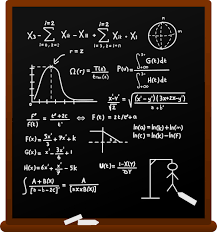# Features in TamStatSimple TamStat expressions can easily be entered in the session.  However, more complex expressions are easier to create using one of the following wizards built into TamStat.

• Summary Wizard - Displays summary statistics for a named variable.  An optional grouping variable may be assigned.
• Probability Wizard - Calculates event probabilities from a probability distribution or contingency table using the rules of probability.  Display Venn Diagram graphics.
• Distribution Wizard - Calculate probability, critical value or theoretical values for any distribution.  Also generate random variables from any distributions.   Optional graphics.
• Statistical Tables - Build standard statistical tables including Binomial, Normal, t, F and Chi-Square.  Users may also generate custom tables.
• Confidence Intervals - Find confidence intervals for various parameters using several confidence levels.    Create comparison graphics.
• Hypothesis Wizard - Perform a one- or two-sample hypothesis test for various parameters and generate a report.
• Sample Size Calculator - Find the minimum sample size for estimating means and proportions.
• ANOVA - Perform a one-way or two-way Analysis of Variance test
• Regression Wizard - Perform a least-squares estimate to calculate the intercept and slope(s) for simple or multiple linear regression.  Generate a report including R-Squared, standard error and other calculations.
• Chi-Square Tests - Perform tests of independence and goodness of fit.
• Bayesian Analysis - Calculate posterior probabilities given prior and and conditional probabilities.